# Technical Drawing Visual Communication of Technical Information Technical

• Slides: 23Technical Drawing Visual Communication of Technical InformationTechnical Drawing Visual Communication of Technical Information n Unit Overview – several classes Precise Drawings Give More Information Types of Technical Drawing n n n Isometric Projections Parallel, Perspective, and Oblique Orthographic Projections Multi-view Sketches Practice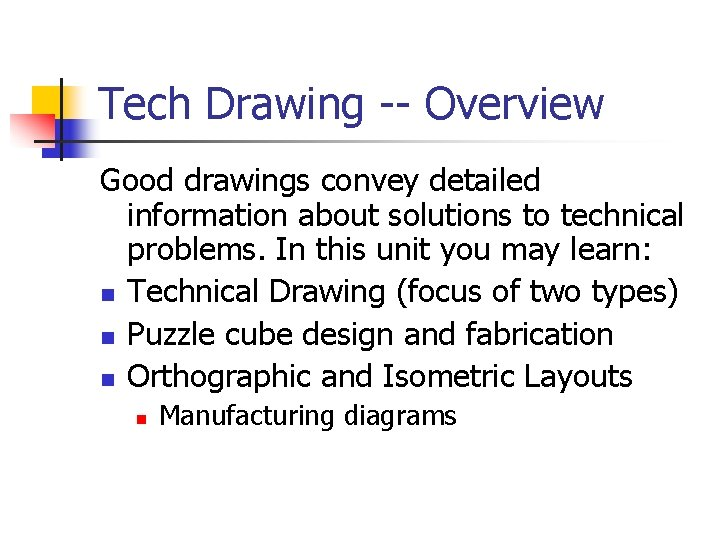Tech Drawing -- Overview Good drawings convey detailed information about solutions to technical problems. In this unit you may learn: n Technical Drawing (focus of two types) n Puzzle cube design and fabrication n Orthographic and Isometric Layouts n Manufacturing diagramsHow can we present an object and learn the most about it? This view shows a square object with a number and some other detail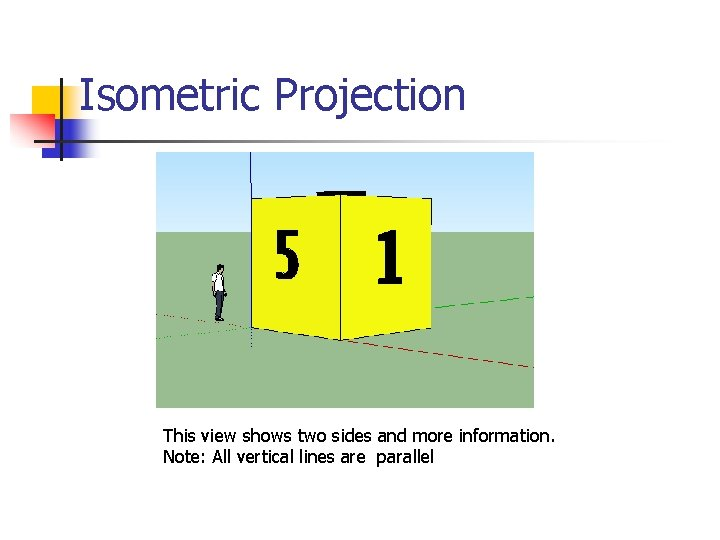Isometric Projection This view shows two sides and more information. Note: All vertical lines are parallelIsometric Projection This view shows three sides and even more information. All vertical lines are parallel; all other lines are 30 to 60 degrees above the horizon.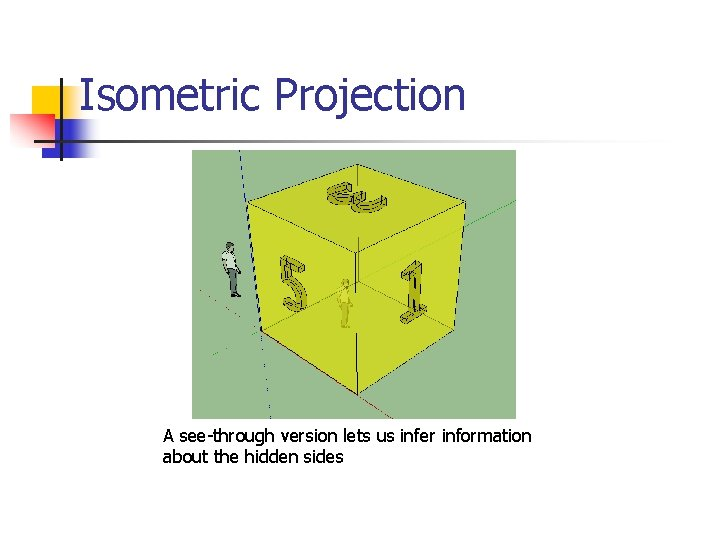Isometric Projection A see-through version lets us infer information about the hidden sides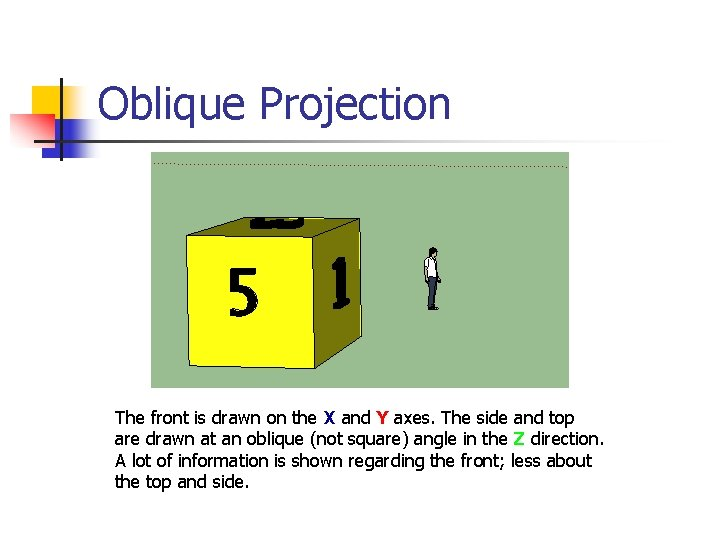Oblique Projection The front is drawn on the X and Y axes. The side and top are drawn at an oblique (not square) angle in the Z direction. A lot of information is shown regarding the front; less about the top and side.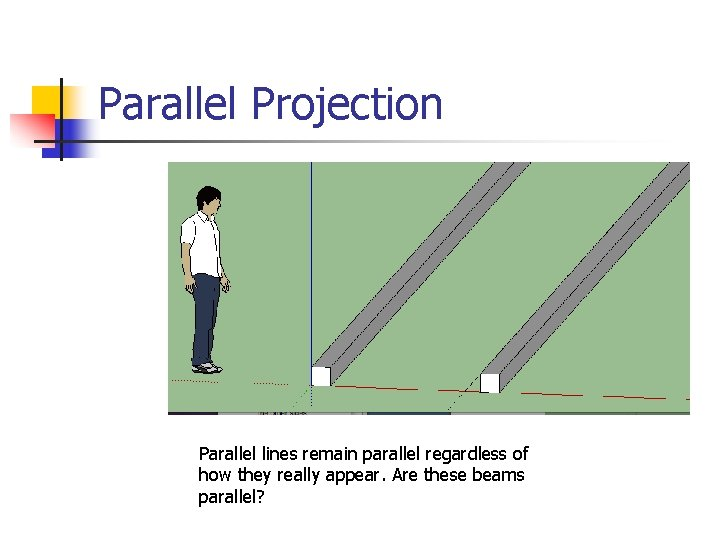Parallel Projection Parallel lines remain parallel regardless of how they really appear. Are these beams parallel?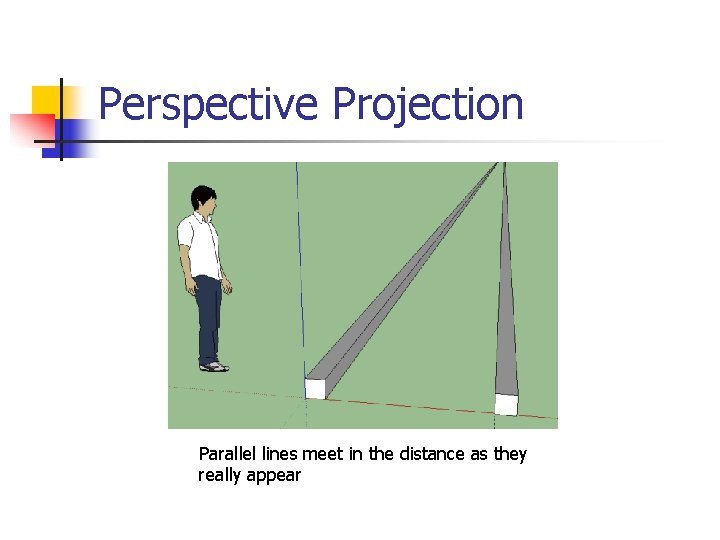Perspective Projection Parallel lines meet in the distance as they really appearPerspective and Parallel The cube is scaled to be 12 ft.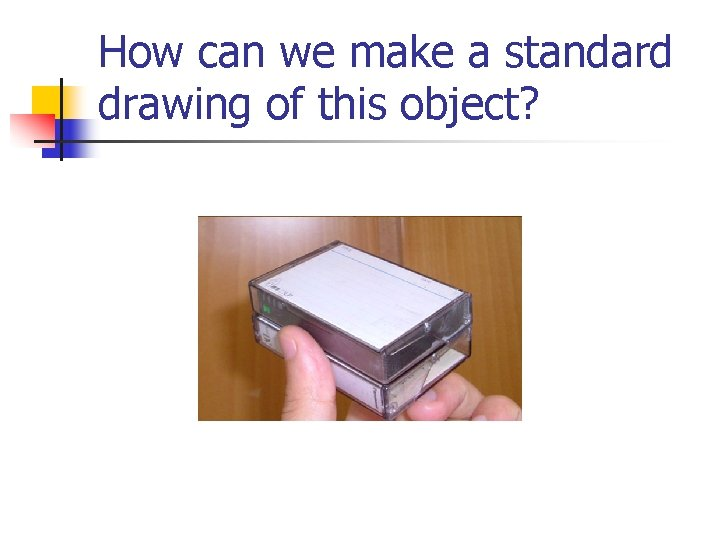How can we make a standard drawing of this object?To begin: All vertical lines are perpendicular to the horizon HorizonNext: All other lines rise at 30 degrees from the horizon Horizon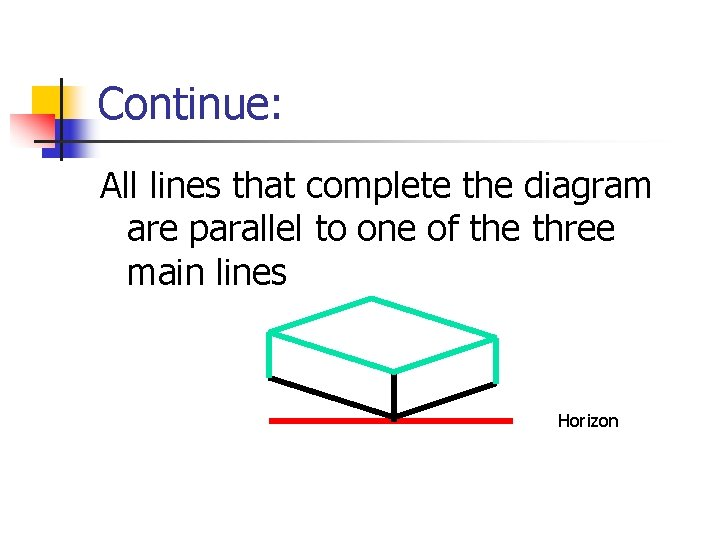Continue: All lines that complete the diagram are parallel to one of the three main lines Horizon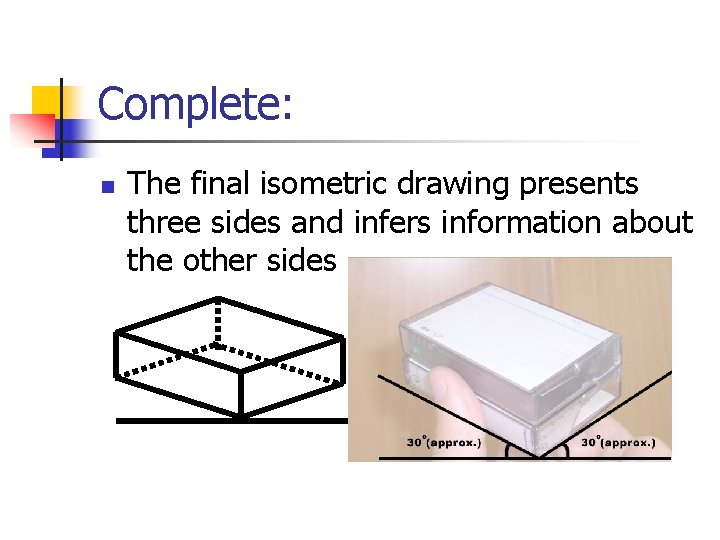Complete: n The final isometric drawing presents three sides and infers information about the other sides Horizon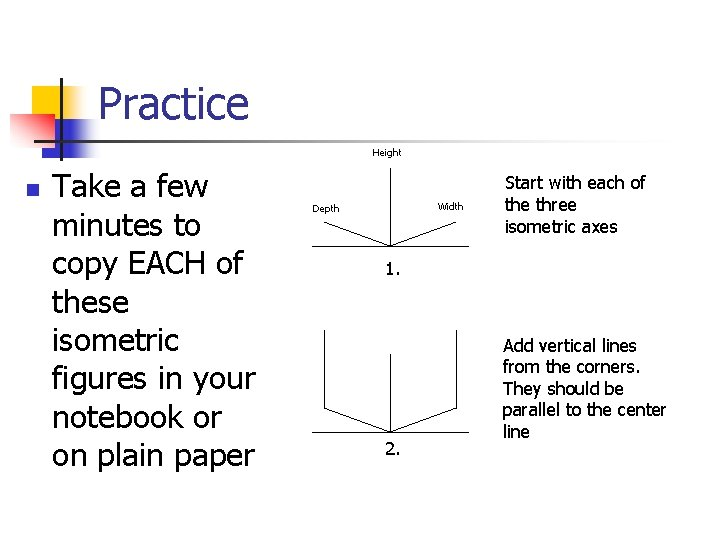Practice Height n Take a few minutes to copy EACH of these isometric figures in your notebook or on plain paper Width Depth Start with each of the three isometric axes 1. 2. Add vertical lines from the corners. They should be parallel to the center line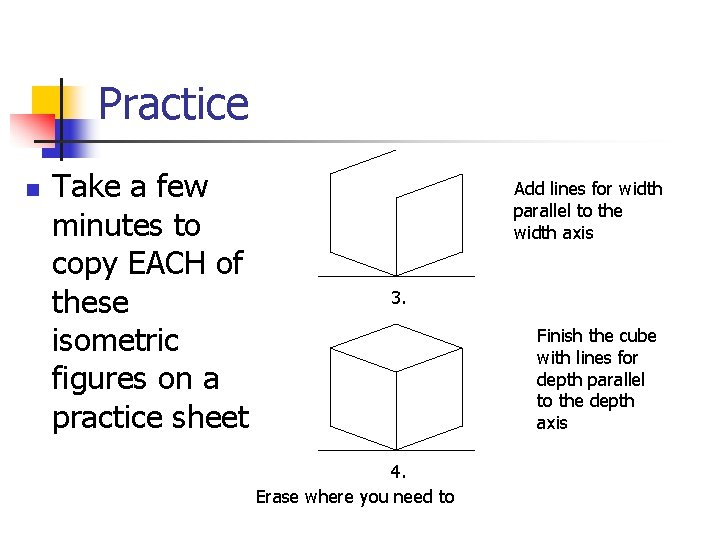Practice n Take a few minutes to copy EACH of these isometric figures on a practice sheet Add lines for width parallel to the width axis 3. Finish the cube with lines for depth parallel to the depth axis 4. Erase where you need toPractice n Draw an isometric sketch of the boxes in the diagram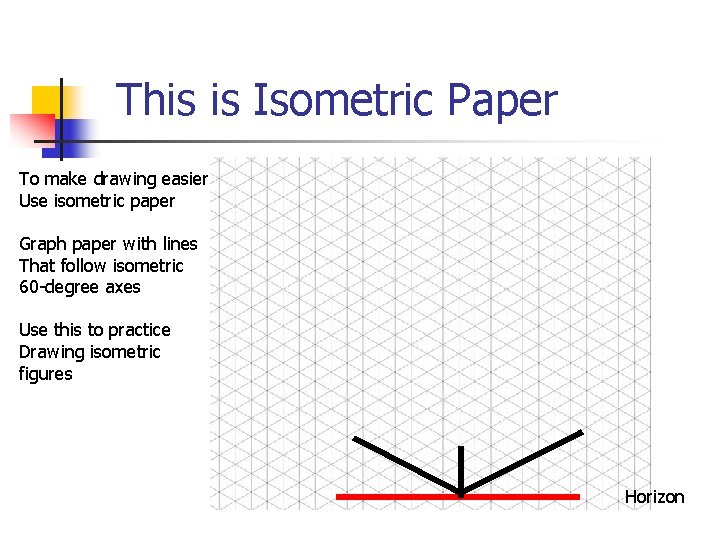This is Isometric Paper To make drawing easier Use isometric paper Graph paper with lines That follow isometric 60 -degree axes Use this to practice Drawing isometric figures HorizonMore Practice There are seven 3 -D figures made of cubes on your work sheet. Construct each using plastic cubes. `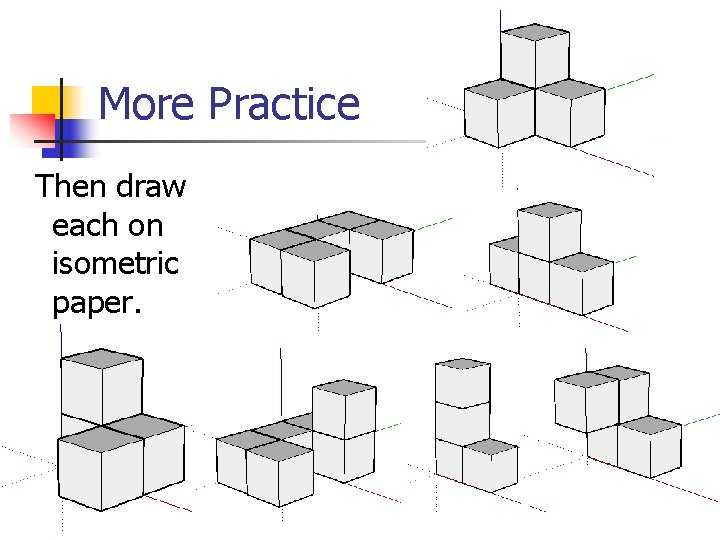More Practice Then draw each on isometric paper.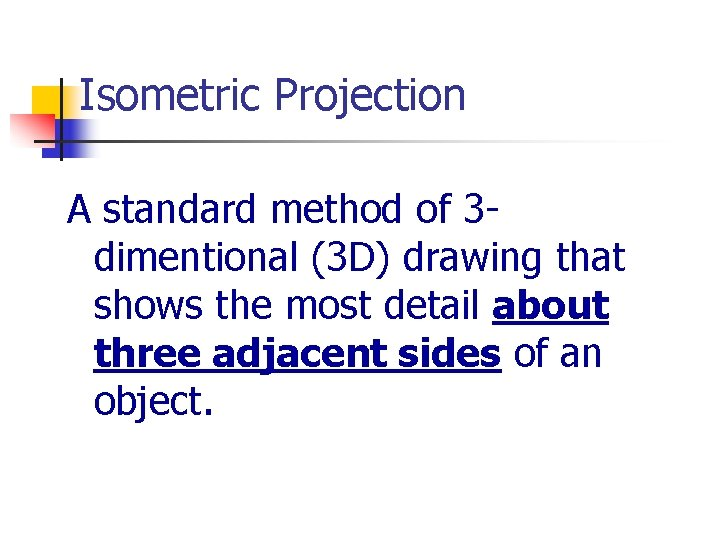Isometric Projection A standard method of 3 dimentional (3 D) drawing that shows the most detail about three adjacent sides of an object.